##### The Japanese Bonsai specialist
Direct order Contact Help / Services Newsletter# Juniperus chinensis itoigawa ref :1907196

Juniperus chinensis itoigawa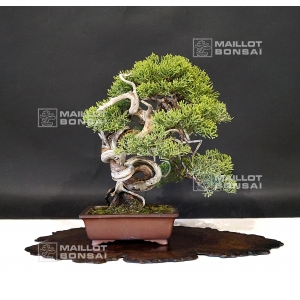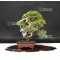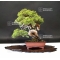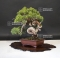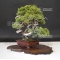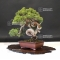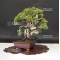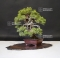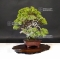ref. : 101891.584,00

Available quantity : 1Order

###### Description

Height of the tree without its pot: 220 mm. Width of the branches: 220 mm.

Artisanal quality non-enamelled pot:  137*112*45 mm. Moyogi style.

Juniper with graceful trays, a shari on its twisted trunk and jins.

Itoigawa variety with thin green canopy scales, particularly vigorous and perfectly adapted to the bonsaï cultivation.

Unlike the classic junieprus chinensis, it only loses little foliage in the beginning of summer. This subject is more than 40/45 so its bark is formed, coming from natural yamadori.

any strong wire wound nor cutting wound.

Remarkable subject! Authentic bonsaï with natural jin and shari, do not mistake with tanukis.

Reshaped in February 2019 with copper wire by Master Tomoya Nishikawa.

Coming from Master Shiraichi's nursery.

The front face was softly modified and moved to the right (more than in the photos) for the next time it's repoted, so that you can see the knotted trunk that makes that bonsaï unique.

Do you have this complete book about juniper?

Shelf non-included. Photographed in July 2019.

#bonsai 5.6 #with 5.4 #chinensis 3.9 #itoigawa 3.8 #that 3.4 #coniferous 3 #juniperus 2.9 #natural 2.7 #coming 2.6 #master 2.6

Formule
(( ROUND((CHAR_LENGTH(b.article_nom)-CHAR_LENGTH(REPLACE(b.article_nom, 'with', '')))/LENGTH('with')) + ROUND((CHAR_LENGTH(b.article_description)-CHAR_LENGTH(REPLACE(b.article_description, 'with', '')))/LENGTH('with')) ) * 5.4) + (( ROUND((CHAR_LENGTH(b.article_nom)-CHAR_LENGTH(REPLACE(b.article_nom, 'chinensis', '')))/LENGTH('chinensis')) + ROUND((CHAR_LENGTH(b.article_description)-CHAR_LENGTH(REPLACE(b.article_description, 'chinensis', '')))/LENGTH('chinensis')) ) * 3.9) + (( ROUND((CHAR_LENGTH(b.article_nom)-CHAR_LENGTH(REPLACE(b.article_nom, 'itoigawa', '')))/LENGTH('itoigawa')) + ROUND((CHAR_LENGTH(b.article_description)-CHAR_LENGTH(REPLACE(b.article_description, 'itoigawa', '')))/LENGTH('itoigawa')) ) * 3.8) + (( ROUND((CHAR_LENGTH(b.article_nom)-CHAR_LENGTH(REPLACE(b.article_nom, 'bonsai', '')))/LENGTH('bonsai')) + ROUND((CHAR_LENGTH(b.article_description)-CHAR_LENGTH(REPLACE(b.article_description, 'bonsai', '')))/LENGTH('bonsai')) ) * 3.6) + (( ROUND((CHAR_LENGTH(b.article_nom)-CHAR_LENGTH(REPLACE(b.article_nom, 'that', '')))/LENGTH('that')) + ROUND((CHAR_LENGTH(b.article_description)-CHAR_LENGTH(REPLACE(b.article_description, 'that', '')))/LENGTH('that')) ) * 3.4) + (( ROUND((CHAR_LENGTH(b.article_nom)-CHAR_LENGTH(REPLACE(b.article_nom, 'juniperus', '')))/LENGTH('juniperus')) + ROUND((CHAR_LENGTH(b.article_description)-CHAR_LENGTH(REPLACE(b.article_description, 'juniperus', '')))/LENGTH('juniperus')) ) * 2.9) + (( ROUND((CHAR_LENGTH(b.article_nom)-CHAR_LENGTH(REPLACE(b.article_nom, 'natural', '')))/LENGTH('natural')) + ROUND((CHAR_LENGTH(b.article_description)-CHAR_LENGTH(REPLACE(b.article_description, 'natural', '')))/LENGTH('natural')) ) * 2.7) + (( ROUND((CHAR_LENGTH(b.article_nom)-CHAR_LENGTH(REPLACE(b.article_nom, 'coming', '')))/LENGTH('coming')) + ROUND((CHAR_LENGTH(b.article_description)-CHAR_LENGTH(REPLACE(b.article_description, 'coming', '')))/LENGTH('coming')) ) * 2.6) + (( ROUND((CHAR_LENGTH(b.article_nom)-CHAR_LENGTH(REPLACE(b.article_nom, 'master', '')))/LENGTH('master')) + ROUND((CHAR_LENGTH(b.article_description)-CHAR_LENGTH(REPLACE(b.article_description, 'master', '')))/LENGTH('master')) ) * 2.6) + (( ROUND((CHAR_LENGTH(b.article_nom)-CHAR_LENGTH(REPLACE(b.article_nom, 'wound', '')))/LENGTH('wound')) + ROUND((CHAR_LENGTH(b.article_description)-CHAR_LENGTH(REPLACE(b.article_description, 'wound', '')))/LENGTH('wound')) ) * 2.5)

## Secure payment## Delivery

Our logistic partners :04 74 55 23 48
Pépinière MAILLOT-BONSAÏ
Le Bois Frazy
01990 RELEVANT - FRANCE
on appointment